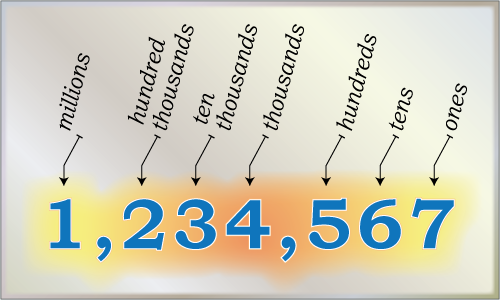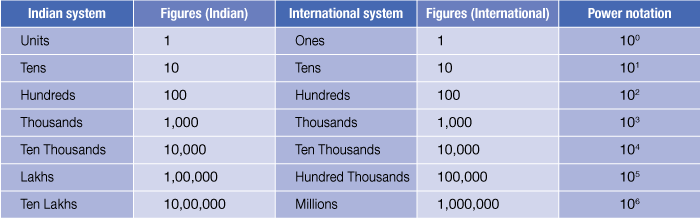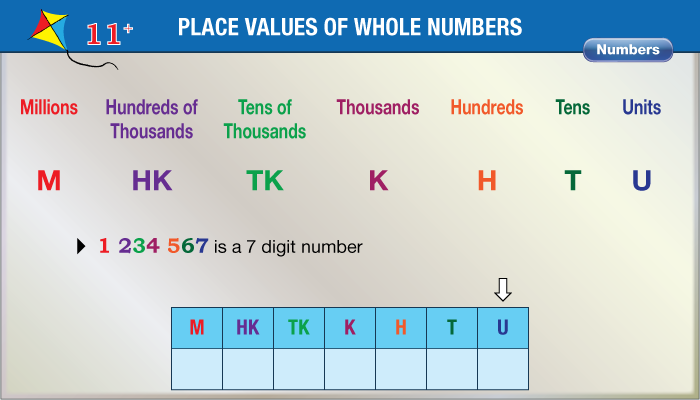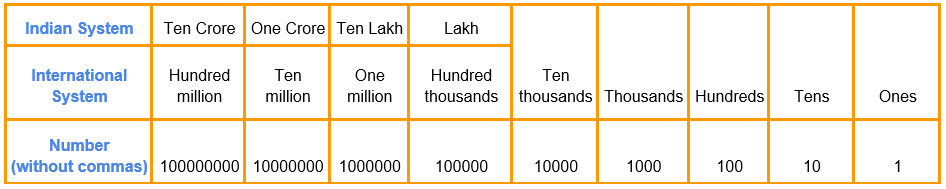# Numbers up to 7-Digits

Go back to  'Numbers and Place values'

## Introduction to Numbers up to 7-Digits

You can easily visualize a dozen eggs (two-digit numbers), and a cricketer scoring a century ($$3$$ digits). You can think of the number of minutes in a day ($$1440$$) as a $$4$$-digit number. But for the number of seconds in a day, you would need a $$5$$-digit number ($$86400$$). The next big jump is to try and visualize $$6$$-digit numbers, and here you would come into the realm of lakhs. Maybe the population of a particular area of a city could be in $$6$$ digits. But when it comes to the population of metropolitan cities, you would need $$7$$ digits to describe it, which means you would get introduced to the word million. The word million is written as the digit followed by $$6$$ zeroes and is the smallest $$7$$ digit word.## The Big Idea: Numbers up to 7-Digits

### A simple idea: The Use of Commas

$$7$$ digit ones are the first group of numbers which can be written in both the Hindu Arabic (Indian) style as well as the international style. While learning about $$6$$ digit numbers, we did discuss how the commas are used in sets of $$3:2:2$$ in the Indian numbering style. So for the $$6$$ digit number $$345783,$$ the Indian system would have it written as $$3,45,783.$$

Let us move on to the $$7$$ digit world of millions now, and take a random $$7$$-digit number $$5290329.$$ This number would have two ways of expression in both the systems. In the familiar Indian system of numbers, you would write this number as $$52,90,329$$ and would express it as $$52$$ lakhs ninety thousand three hundred and twenty-nine.The international numbering system introduces the word million, where a million is equal to $$10$$ lakhs. $$1$$ million $$= 10,00,000.$$

But the commas in the above number are placed as per the Indian $$2:2:3$$ system. The international system uses the $$3:3$$ system, so the way $$1$$ million would be written in the international system is $$1$$ million $$= 10,00,000.$$

Did you notice how the same number of $$10$$ lakhs (or $$1$$ million) looks different in both the systems? In the Indian system the lakh begins after the first five digits from the right; therefore the comma after the first five digits from right tells us how many lakhs there are (in this case $$52$$). In the international system, the million begins after the six digits from the right, so the comma after the sixth digit from right signifies how many millions there are. If we take the same number, we would write it as $$5,290,329$$ and understand that the number is $$5$$ million, instead of saying $$52$$ lakhs.

### Place Values In 7-digit numbers

Till the six-digit numbers, we saw the place values of lakhs, apart from the earlier place value of ten thousands, thousands, hundreds, tens and units. When we come to $$7$$-digit numbers, the next place value added is ten lakhs. As we explained above, this place value could also be expressed as millions.### How To Decompose 7-digit numbers

Any seven digit number has place values up to ten lakh. These are the names of the place values (starting from the right) in a seven digit number, as shown in the picture above:

• Digit $$1$$ – Units
• Digit $$2$$ – Tens
• Digit $$3$$ – Hundreds
• Digit $$4$$ – Thousands
• Digit $$5$$ – Ten Thousands
• Digit $$6$$ – Lakhs
• Digit $$7$$ – Ten Lakhs / Millions

So let us take the seven digit number we discussed above - $$5290329,$$ and see how it gets decomposed.

\begin{align} &{ \text { Digit } 1 \text { Place Value} = 9 \times 1 = \qquad \qquad \quad \;\;\;\, 0} \\ &{ \text { Digit } 2 \text { Place Value} = 2 \times 10 = \qquad \qquad \;\;\;\, 20} \\ &{ \text { Digit } 3 \text { Place Value} = 3 \times 100 = \qquad \quad \;\;\;\, 300} \\& { \text { Digit } 4 \text { Place Value} = 0 \times 1000 = \quad \quad \;\;\;\, 0000 } \\ &{ \text { Digit } 5 \text { Place Value} = 0 \times 10000 = \quad \;\;\;\, 90000 } \\ &{ \text { Digit } 6 \text { Place Value} = 2 \times 100000 = \;\;\;\,200000 } \\ &{ \text { Digit } 7 \text { Place Value} = 5 \times 1000000 = 5000000 } \end{align}

## How is it important?

### Zeroes in 7-digit numbers

There are $$7$$ place values in every $$7$$-digit number, and any number from $$0$$ to $$9$$ can be put into any of these place values. There is only one exception. If the leftmost place value (millions / Ten Lakhs) is zero, then it becomes a $$6$$-digit number. If the digits in $$5290329$$ are rearranged to place the zero on the extreme left, the number becomes $$0295329,$$ in which case it becomes the $$6$$-digit number $$295329.$$

## Common mistakes or misconceptions

• Misconception: One million” and “ten lakh” are two different numbers.
In India, children are taught to name numbers based on both the Indian naming system and the International naming system. So, 1,000,000 is written as “One million” as per the International naming system but “Ten lakh” as per the Indian system.
• Children tend to make mistakes when placing commas in numbers of 7 or more digits.
This once again happens because of two conventions - the Indian system and the International system. Here children should build comfort writing the same number in both systems. Targeted practice helps.

## Tips and Tricks

Tip: Create a chart with all the place names listed. Let children see how till ten-thousand the naming convention is the same. But for 7 digits or higher the same number has two names. The choice of names does not change the value of the number. “One lakh” and “one hundred thousand” are equal (have the same value).Tip: Remember the number of zeros after 1 for two large numbers. E.g. remember that “one crore” is 1 followed by 7 zeroes. And “1 million” is 1 followed by 6 zeroes. All other larger or smaller numbers can then be written with reference to these two numbers.

Trick: Children tend to make mistakes when rounding off a number. If we want to round off 4,63,859 to the nearest thousand, it helps to simply underline all digits that are in the lower place values (ones, tens and hundreds, in this example). Then simply replace those digits with 0. While doing this check the highest place value we are replacing - hundreds place in this case. If that is 5 or higher increase the thousands place by one. So the rounded off number will become 4,64,000.